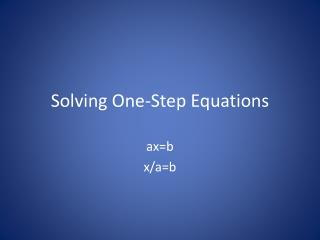Download PresentationSolving One-Step Equations

# Solving One-Step Equations - PowerPoint PPT PresentationDownload Presentation## Solving One-Step Equations

- - - - - - - - - - - - - - - - - - - - - - - - - - - E N D - - - - - - - - - - - - - - - - - - - - - - - - - - -
##### Presentation Transcript

1. Solving One-Step Equations ax=b x/a=b

2. Solve equations by “isolating the variable” • Get the variable by itself • We do this by using opposite operations • The opposite of addition is subtraction • The opposite of multiplication is division • ALWAYS SHOW YOUR WORK!!!!!!

3. Examples • 3x = 24 - x is being multiplied by 3, so we must divide by 3 to get x by itself - Whatever you do to one side, do the same to the other side 3x/3 = 24/3 x = 8 CHECK: 3(8) = 24 24 = 24

4. r/2 = 14 - r is being divided by 2, so we must multiply by 2 to get r by itself - r/2 × 2 = 14 × 2 - r = 28 CHECK: 28/2 = 14 14 = 14

5. -3t = -36 4. n/3 = -7 • -5b = 45 6. d/-5 = 3

6. Word Problems • ⅙ of Canada’s planetariums are in Winnipeg. There are 2 planetariums in Winnipeg. How many planetariums are in Canada? - Assign a variable - p = # of planetariums in Canada - p/6 = 2

7. The average mass of a lynx is 16 kg. This mass is twice the mass of an otter. Calculate the mass of an otter. - o = otter’s mass - 2o = 16

8. Assignment • Pg 377 – 379 # 11 – 26